# Selina Solutions Concise Maths Class 10 Chapter 12 Reflection

This Chapter gives a brief on co-ordinate geometry and other important concepts like the reflection of a point in different co-ordinate axes, origin and with respect to a line. It is very important for the students to have strong command about the concepts covered in each chapter of Class 10 as it’s a turning point in a student’s life. To make the process easier, BYJU’S has created the Selina Solutions for Class 10 Mathematics which is a valuable resource for students. After all, it’s advantageous to obtain a good score in a subject like Mathematics. The solutions are made available in PDF format so that the students can download it. The Selina Solutions for Class 10 Mathematics Chapter 12 Reflection PDF are given below.

## Selina Solutions Concise Maths Class 10 Chapter 12 Reflection Download PDF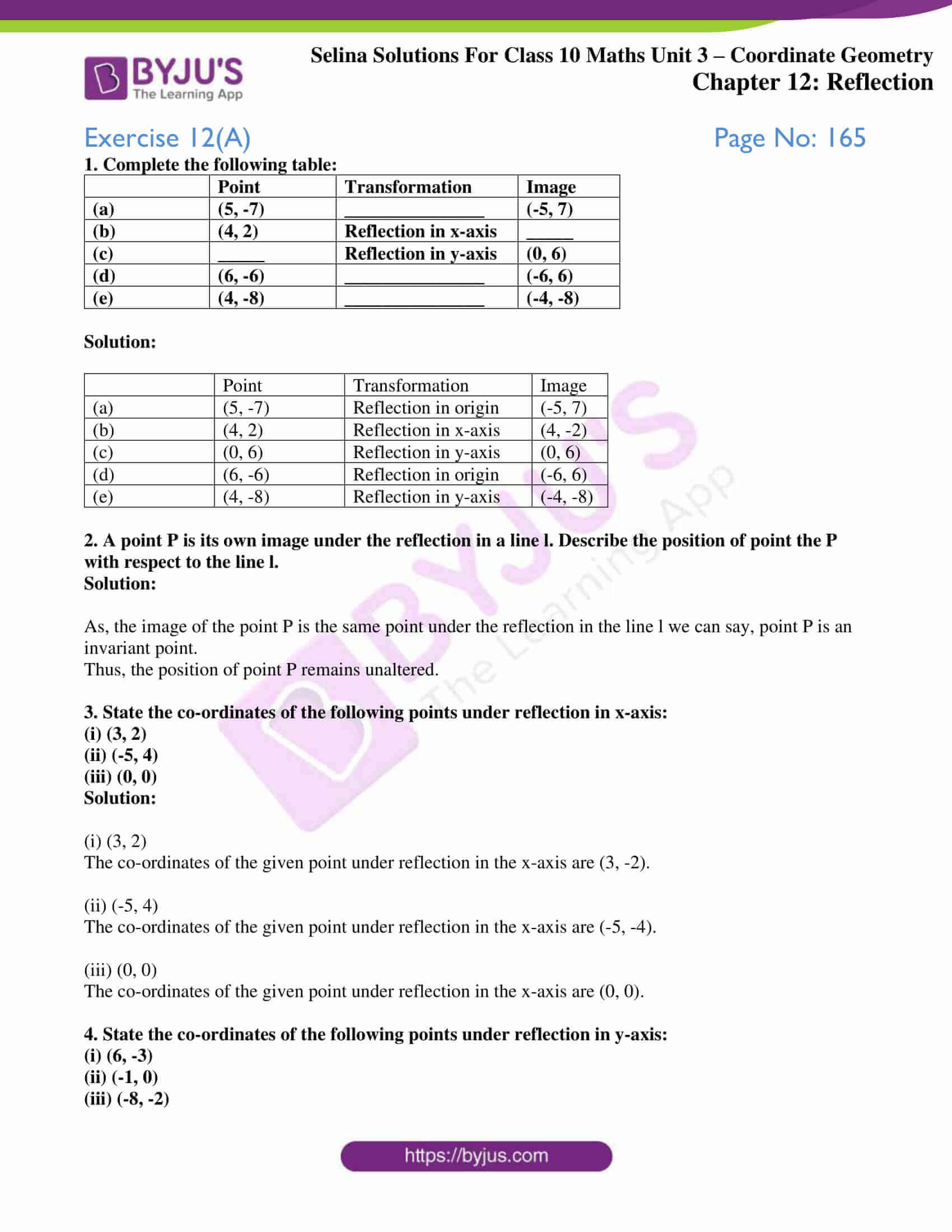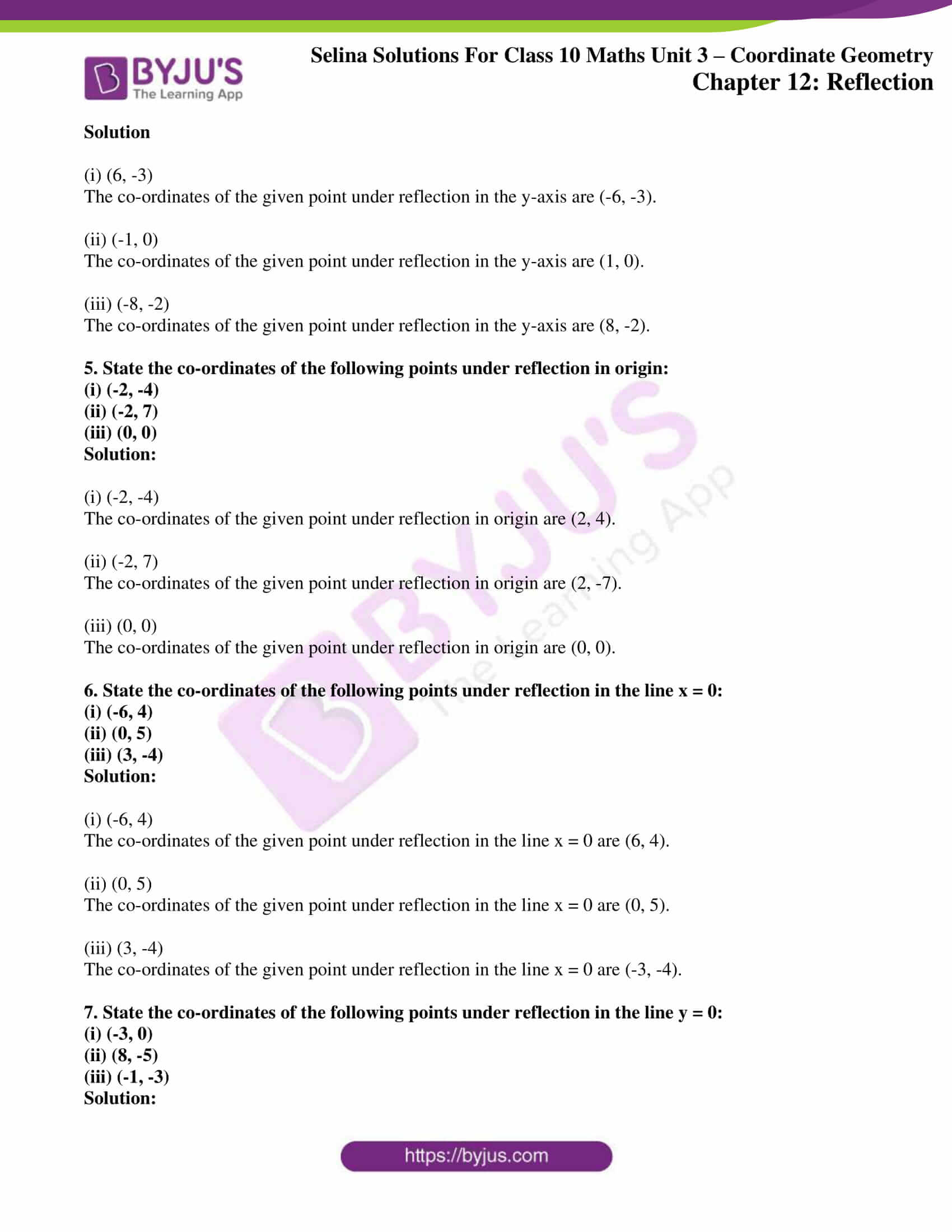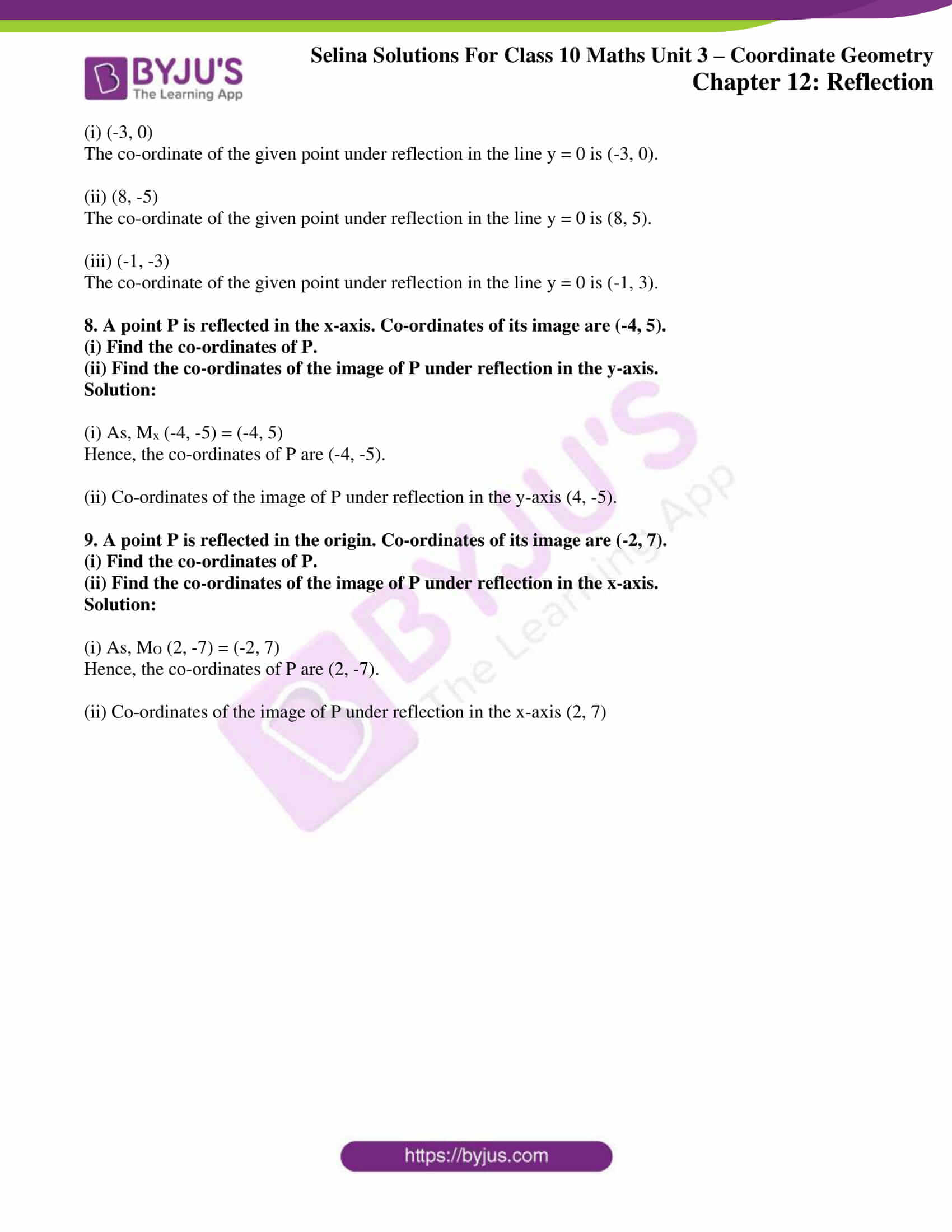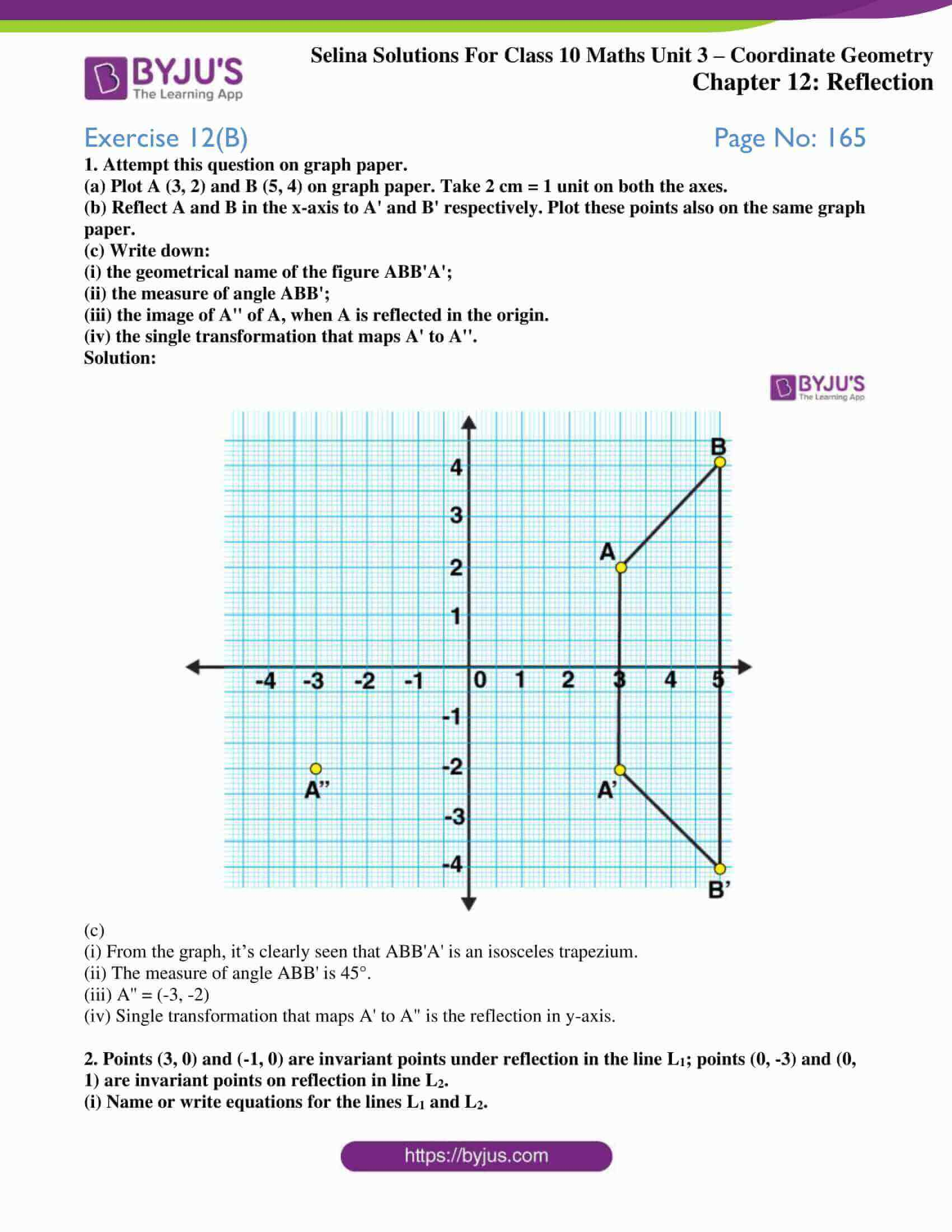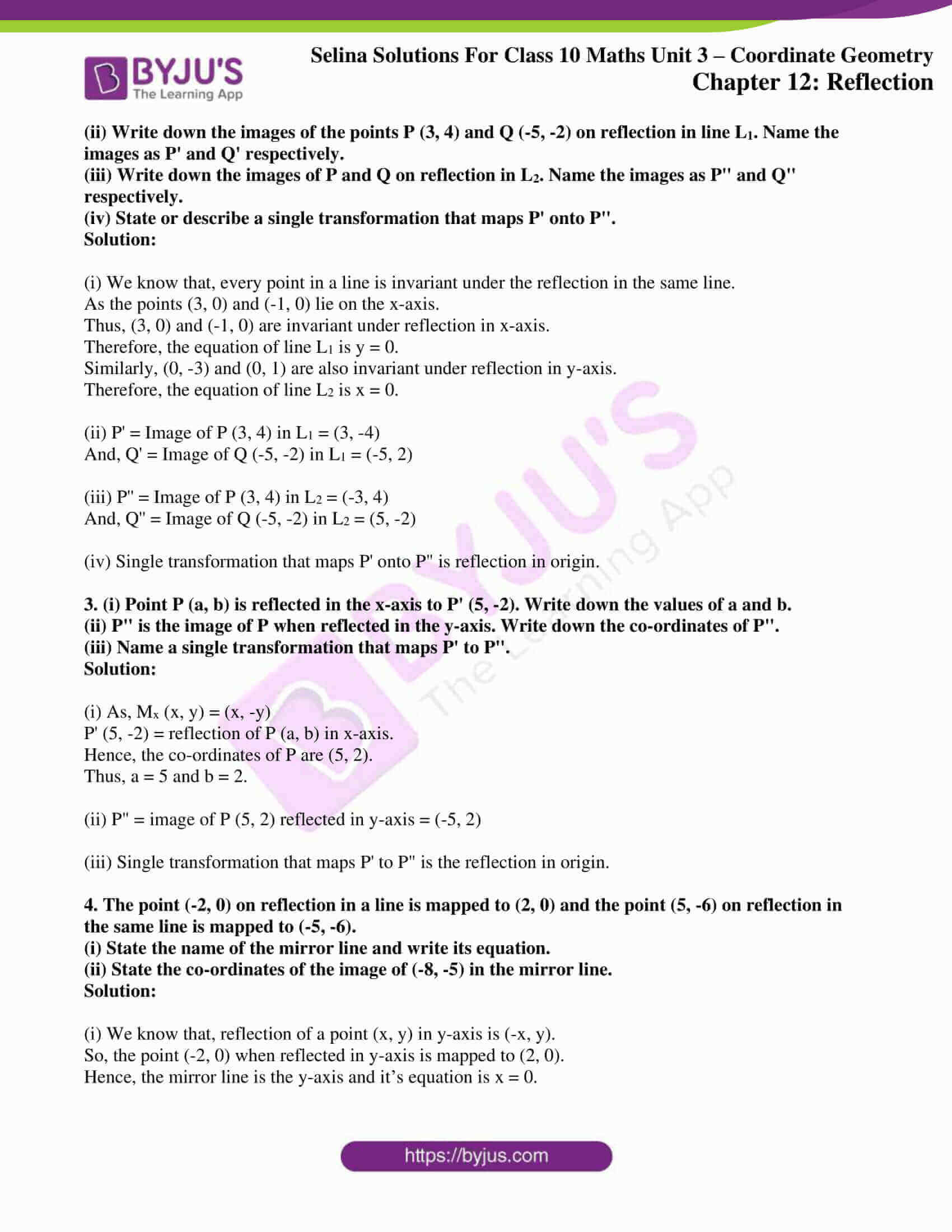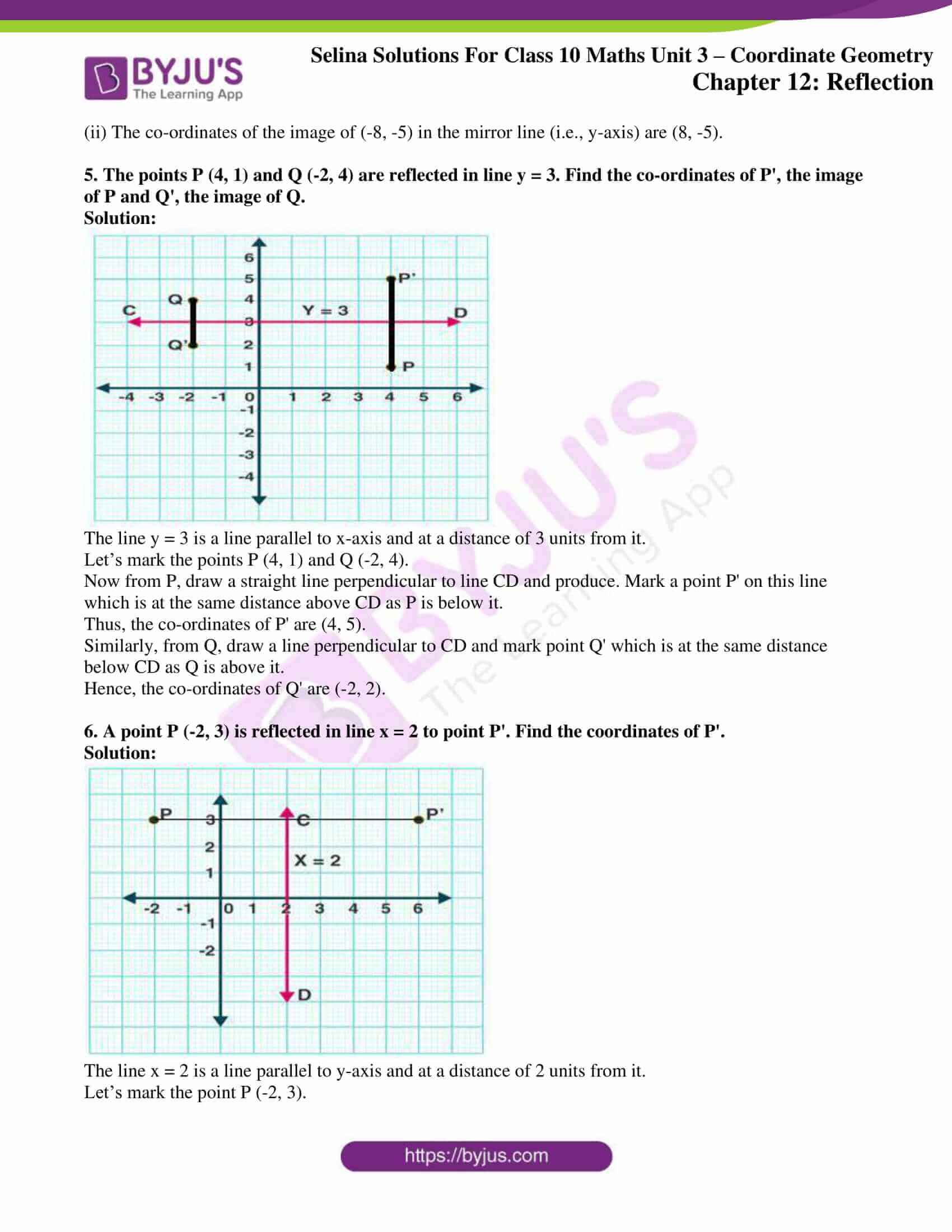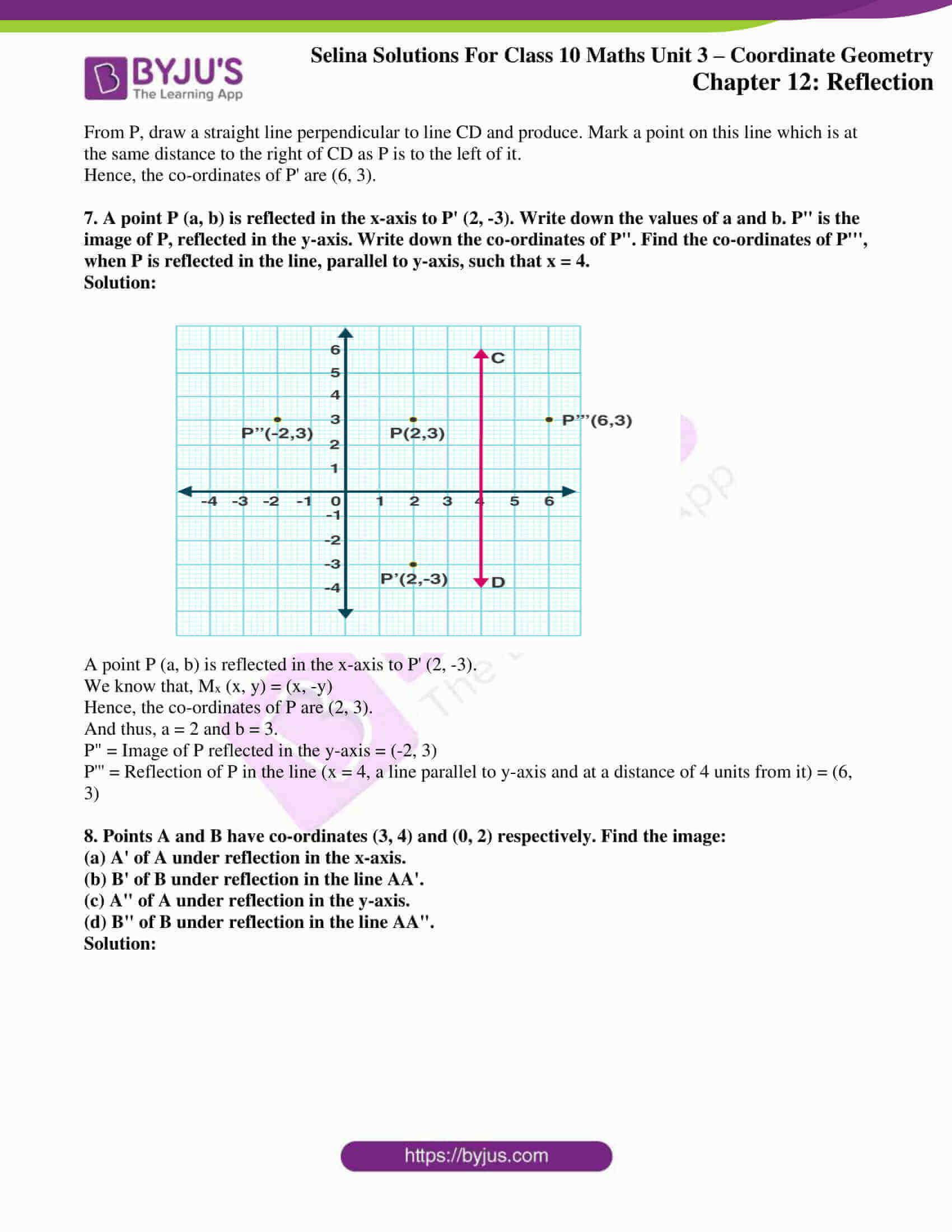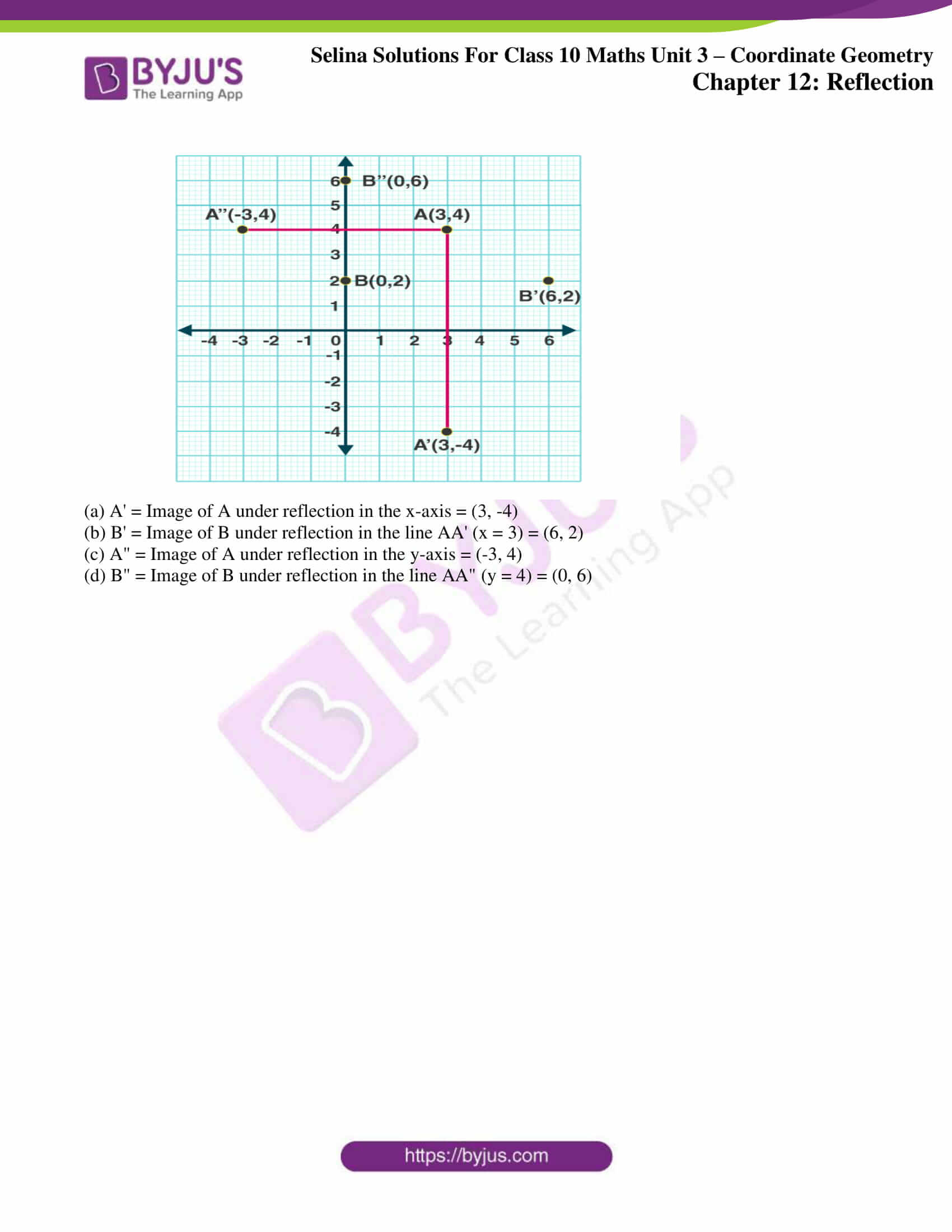### Exercises of Selina Solutions Concise Maths Class 10 Chapter 12 Reflection

Exercise 12(A) Solutions

Exercise 12(B) Solutions

## Access Selina Solutions Concise Maths Class 10 Chapter 12 Reflection

Exercise 12(A) Page No: 165

1. Complete the following table:

 Point Transformation Image (a) (5, -7) _______________ (-5, 7) (b) (4, 2) Reflection in x-axis _____ (c) _____ Reflection in y-axis (0, 6) (d) (6, -6) _______________ (-6, 6) (e) (4, -8) _______________ (-4, -8)

Solution:

 Point Transformation Image (a) (5, -7) Reflection in origin (-5, 7) (b) (4, 2) Reflection in x-axis (4, -2) (c) (0, 6) Reflection in y-axis (0, 6) (d) (6, -6) Reflection in origin (-6, 6) (e) (4, -8) Reflection in y-axis (-4, -8)

2. A point P is its own image under the reflection in a line l. Describe the position of point the P with respect to the line l.

Solution:

As, the image of the point P is the same point under the reflection in the line l we can say, point P is an invariant point.

Thus, the position of point P remains unaltered.

3. State the co-ordinates of the following points under reflection in x-axis:

(i) (3, 2)

(ii) (-5, 4)

(iii) (0, 0)

Solution:

(i) (3, 2)

The co-ordinates of the given point under reflection in the x-axis are (3, -2).

(ii) (-5, 4)

The co-ordinates of the given point under reflection in the x-axis are (-5, -4).

(iii) (0, 0)

The co-ordinates of the given point under reflection in the x-axis are (0, 0).

4. State the co-ordinates of the following points under reflection in y-axis:

(i) (6, -3)

(ii) (-1, 0)

(iii) (-8, -2)

Solution

(i) (6, -3)

The co-ordinates of the given point under reflection in the y-axis are (-6, -3).

(ii) (-1, 0)

The co-ordinates of the given point under reflection in the y-axis are (1, 0).

(iii) (-8, -2)

The co-ordinates of the given point under reflection in the y-axis are (8, -2).

5. State the co-ordinates of the following points under reflection in origin:

(i) (-2, -4)

(ii) (-2, 7)

(iii) (0, 0)

Solution:

(i) (-2, -4)

The co-ordinates of the given point under reflection in origin are (2, 4).

(ii) (-2, 7)

The co-ordinates of the given point under reflection in origin are (2, -7).

(iii) (0, 0)

The co-ordinates of the given point under reflection in origin are (0, 0).

6. State the co-ordinates of the following points under reflection in the line x = 0:

(i) (-6, 4)

(ii) (0, 5)

(iii) (3, -4)

Solution:

(i) (-6, 4)

The co-ordinates of the given point under reflection in the line x = 0 are (6, 4).

(ii) (0, 5)

The co-ordinates of the given point under reflection in the line x = 0 are (0, 5).

(iii) (3, -4)

The co-ordinates of the given point under reflection in the line x = 0 are (-3, -4).

7. State the co-ordinates of the following points under reflection in the line y = 0:

(i) (-3, 0)

(ii) (8, -5)

(iii) (-1, -3)

Solution:

(i) (-3, 0)

The co-ordinate of the given point under reflection in the line y = 0 is (-3, 0).

(ii) (8, -5)

The co-ordinate of the given point under reflection in the line y = 0 is (8, 5).

(iii) (-1, -3)

The co-ordinate of the given point under reflection in the line y = 0 is (-1, 3).

8. A point P is reflected in the x-axis. Co-ordinates of its image are (-4, 5).

(i) Find the co-ordinates of P.

(ii) Find the co-ordinates of the image of P under reflection in the y-axis.

Solution:

(i) As, Mx (-4, -5) = (-4, 5)

Hence, the co-ordinates of P are (-4, -5).

(ii) Co-ordinates of the image of P under reflection in the y-axis (4, -5).

9. A point P is reflected in the origin. Co-ordinates of its image are (-2, 7).

(i) Find the co-ordinates of P.

(ii) Find the co-ordinates of the image of P under reflection in the x-axis.

Solution:

(i) As, MO (2, -7) = (-2, 7)

Hence, the co-ordinates of P are (2, -7).

(ii) Co-ordinates of the image of P under reflection in the x-axis (2, 7)

Exercise 12(B) Page No: 165

1. Attempt this question on graph paper.

(a) Plot A (3, 2) and B (5, 4) on graph paper. Take 2 cm = 1 unit on both the axes.

(b) Reflect A and B in the x-axis to A’ and B’ respectively. Plot these points also on the same graph paper.

(c) Write down:

(i) the geometrical name of the figure ABB’A’;

(ii) the measure of angle ABB’;

(iii) the image of A” of A, when A is reflected in the origin.

(iv) the single transformation that maps A’ to A”.

Solution: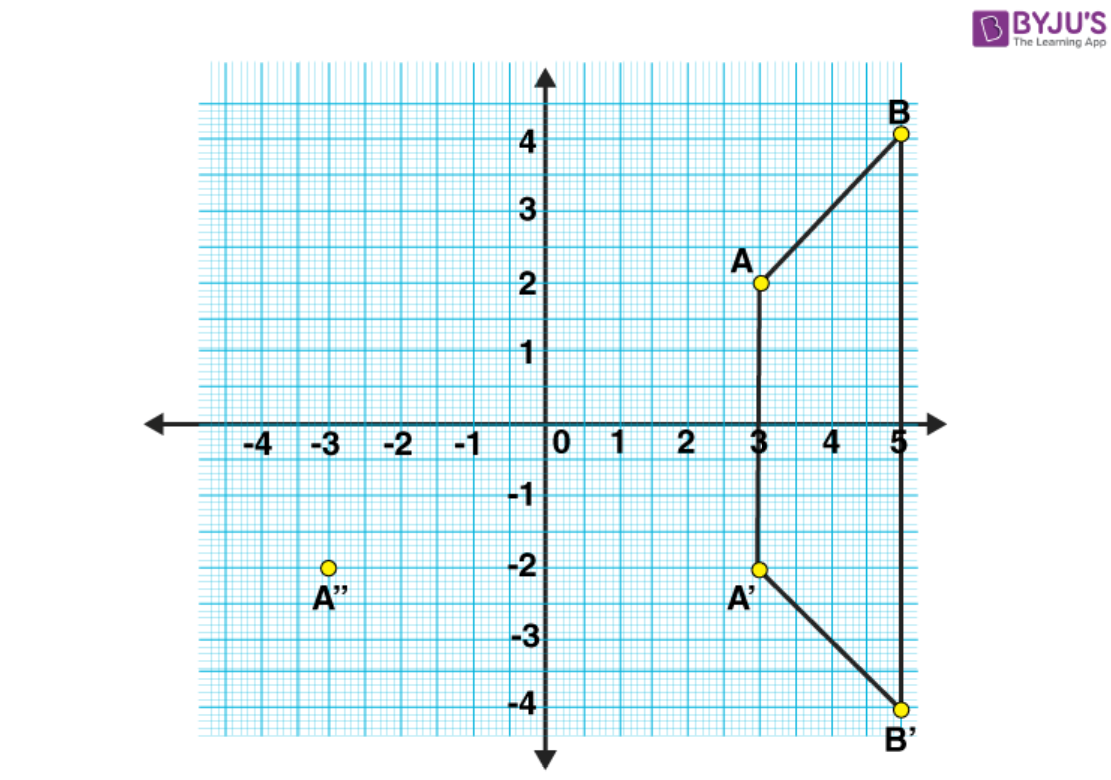(c)

(i) From the graph, it’s clearly seen that ABB’A’ is an isosceles trapezium.

(ii) The measure of angle ABB’ is 45°.

(iii) A” = (-3, -2)

(iv) Single transformation that maps A’ to A” is the reflection in y-axis.

2. Points (3, 0) and (-1, 0) are invariant points under reflection in the line L1; points (0, -3) and (0, 1) are invariant points on reflection in line L2.

(i) Name or write equations for the lines L1 and L2.

(ii) Write down the images of the points P (3, 4) and Q (-5, -2) on reflection in line L1. Name the images as P’ and Q’ respectively.

(iii) Write down the images of P and Q on reflection in L2. Name the images as P” and Q” respectively.

(iv) State or describe a single transformation that maps P’ onto P”.

Solution:

(i) We know that, every point in a line is invariant under the reflection in the same line.

As the points (3, 0) and (-1, 0) lie on the x-axis.

Thus, (3, 0) and (-1, 0) are invariant under reflection in x-axis.

Therefore, the equation of line L1 is y = 0.

Similarly, (0, -3) and (0, 1) are also invariant under reflection in y-axis.

Therefore, the equation of line L2 is x = 0.

(ii) P’ = Image of P (3, 4) in L1 = (3, -4)

And, Q’ = Image of Q (-5, -2) in L1 = (-5, 2)

(iii) P” = Image of P (3, 4) in L2 = (-3, 4)

And, Q” = Image of Q (-5, -2) in L2 = (5, -2)

(iv) Single transformation that maps P’ onto P” is reflection in origin.

3. (i) Point P (a, b) is reflected in the x-axis to P’ (5, -2). Write down the values of a and b.

(ii) P” is the image of P when reflected in the y-axis. Write down the co-ordinates of P”.

(iii) Name a single transformation that maps P’ to P”.

Solution:

(i) As, Mx (x, y) = (x, -y)

P’ (5, -2) = reflection of P (a, b) in x-axis.

Hence, the co-ordinates of P are (5, 2).

Thus, a = 5 and b = 2.

(ii) P” = image of P (5, 2) reflected in y-axis = (-5, 2)

(iii) Single transformation that maps P’ to P” is the reflection in origin.

4. The point (-2, 0) on reflection in a line is mapped to (2, 0) and the point (5, -6) on reflection in the same line is mapped to (-5, -6).

(i) State the name of the mirror line and write its equation.

(ii) State the co-ordinates of the image of (-8, -5) in the mirror line.

Solution:

(i) We know that, reflection of a point (x, y) in y-axis is (-x, y).

So, the point (-2, 0) when reflected in y-axis is mapped to (2, 0).

Hence, the mirror line is the y-axis and it’s equation is x = 0.

(ii) The co-ordinates of the image of (-8, -5) in the mirror line (i.e., y-axis) are (8, -5).

5. The points P (4, 1) and Q (-2, 4) are reflected in line y = 3. Find the co-ordinates of P’, the image of P and Q’, the image of Q.

Solution: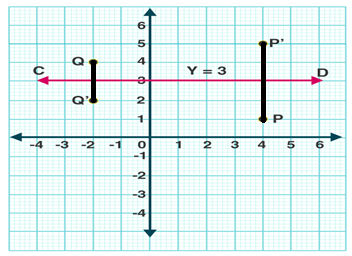The line y = 3 is a line parallel to x-axis and at a distance of 3 units from it.

Let’s mark the points P (4, 1) and Q (-2, 4).

Now from P, draw a straight line perpendicular to line CD and produce. Mark a point P’ on this line which is at the same distance above CD as P is below it.

Thus, the co-ordinates of P’ are (4, 5).

Similarly, from Q, draw a line perpendicular to CD and mark point Q’ which is at the same distance below CD as Q is above it.

Hence, the co-ordinates of Q’ are (-2, 2).

6. A point P (-2, 3) is reflected in line x = 2 to point P’. Find the coordinates of P’.

Solution: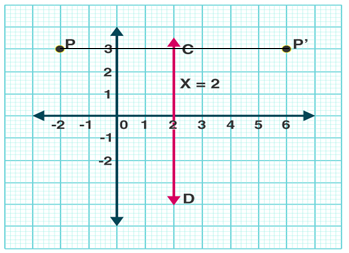The line x = 2 is a line parallel to y-axis and at a distance of 2 units from it.

Let’s mark the point P (-2, 3).

From P, draw a straight line perpendicular to line CD and produce. Mark a point on this line which is at the same distance to the right of CD as P is to the left of it.

Hence, the co-ordinates of P’ are (6, 3).

7. A point P (a, b) is reflected in the x-axis to P’ (2, -3). Write down the values of a and b. P” is the image of P, reflected in the y-axis. Write down the co-ordinates of P”. Find the co-ordinates of P”’, when P is reflected in the line, parallel to y-axis, such that x = 4.

Solution: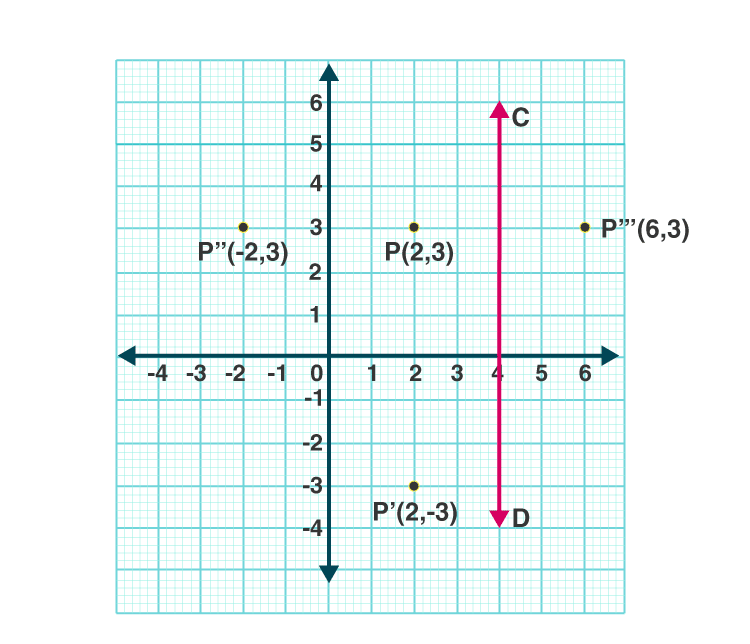A point P (a, b) is reflected in the x-axis to P’ (2, -3).

We know that, Mx (x, y) = (x, -y)

Hence, the co-ordinates of P are (2, 3).

And thus, a = 2 and b = 3.

P” = Image of P reflected in the y-axis = (-2, 3)

P”’ = Reflection of P in the line (x = 4, a line parallel to y-axis and at a distance of 4 units from it) = (6, 3)

8. Points A and B have co-ordinates (3, 4) and (0, 2) respectively. Find the image:

(a) A’ of A under reflection in the x-axis.

(b) B’ of B under reflection in the line AA’.

(c) A” of A under reflection in the y-axis.

(d) B” of B under reflection in the line AA”.

Solution: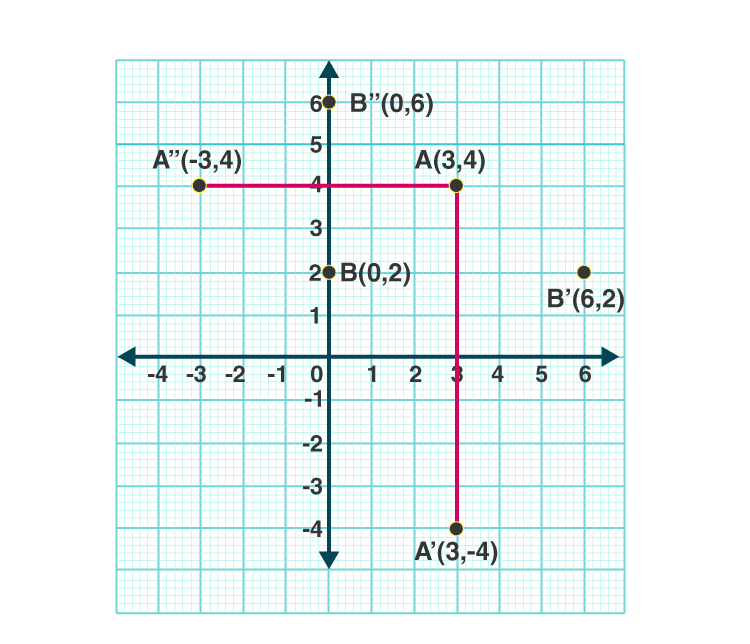(a) A’ = Image of A under reflection in the x-axis = (3, -4)

(b) B’ = Image of B under reflection in the line AA’ (x = 3) = (6, 2)

(c) A” = Image of A under reflection in the y-axis = (-3, 4)

(d) B” = Image of B under reflection in the line AA” (y = 4) = (0, 6)